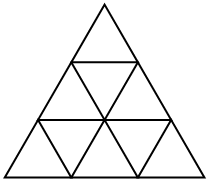## Inscribed triangles and tetrahedra

The following problems appeared in The Riddler. They involve randomly picking points on a circle or sphere and seeing if the resulting shape contains the center or not.

Problem 1: Choose three points on a circle at random and connect them to form a triangle. What is the probability that the center of the circle is contained in that triangle?

Problem 2: Choose four points at random (independently and uniformly distributed) on the surface of a sphere. What is the probability that the tetrahedron defined by those four points contains the center of the sphere?

Here is my solution to both problems:
[Show Solution]

## Counting parallelograms

The following problem appeared in the 1991 CMO, and it has a particularly clever solution.

In the figure, the side length of the large equilateral triangle is $3$ and $f(3)$, the number of parallelograms bounded by sides in the grid, is $15$. For the general analogous situation, find a formula for $f(n)$, the number of parallelograms, for a triangle of side length $n$.[Show Solution]

## A clever integral

I was recently reminded of this problem from one of my favorite books: Problem-Solving Through Problems. The problem originally appeared in the 1980 Putnam Competition.

Evaluate the following definite integral.

$\int_0^{\pi/2} \frac{\mathrm{d}x}{1 + (\tan x)^{\sqrt{2}}}$

The solution:
[Show Solution]